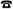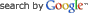DEPT OF ELECTRONIC & ELECTRICAL ENGINEERING LIQUID CRYSTAL MODELLINGINFORMATION FOR

INFORMATION

PROJECTS# Matlab Tips and Tricks

find

Logical indexing:

If you have a vector A, the command A==2 will return a logical vector the same length as A, which takes the value 1 when the entry in A is equal to 2 and 0 otherwise. The following command will replace all occupancies of 2 in a vector with 3.

A(A==2)=3;

Useful commands:

reshape

For sets the following commands are useful

setdiff

union

unique

Interpolation:

interp1

interp2

For matrices the following commands are useful

kron

eye

for instance for the permitivity tensor in terms of the director n is:

epsilonperp*eye(3)+deltaepsilon*kron(n,n');

A = spdiags(vals, [-nx -1:1 nx], nx*nz, nx*nz);

delsqdemo

R = 'L'; % Other possible shapes include S,N,C,D,A,H,B

g = numgrid(R,12)

delsq(g);University College London - Gower Street - London - WC1E 6BT -+44 (0)20 7679 2000 - Copyright © 1999-2005 UCL